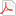Iteration basicsIvarsLong Time FellowPosts: 366 Threads: 26 Joined: Oct 2007 05/24/2008, 08:35 AM bo198214 Wrote:The Abel function counts the iterations of $f$: $\phi(f(f(x)))=\phi(f(x))+1=f(x)+2$ $\phi(f(f(f(x))))=\phi(f(f(x)))+1=f(x)+2+1=f(x)+3$ $\phi(f^{\circ n}(x))=\phi(x)+n$. The fact that Abel function extends COUNTING to non-integer , negative(?) and complex values of COUNTING is very interesting concept besides the fact it is useful in computations and analysis of iterates. $\phi(f^{\circ t}(x))=\phi(x)+t$. e.g. $\phi(f^{\circ I}(x))=\phi(x)+I$. If we turn it upside down, we can may be use the result of t iterations of a function to define what COUNTING with t (e.g. t=I) means. We may get functions which are only COUNTABLE in certain ways, so that Abel equation is not possible to solve for all t. Would that make sense? Ivarsbo198214Administrator Posts: 1,624 Threads: 103 Joined: Aug 2007 05/24/2008, 09:04 AM Ivars Wrote:$\phi(f^{\circ I}(x))=\phi(x)+I$. If we turn it upside down, we can may be use the result of t iterations of a function to define what COUNTING with t (e.g. t=I) means. Ivars, we do exactly that! How do you think we compute $b(I*t)$? I wrote it already bo198214 Wrote:The equation $f^{\circ n}(x)=\phi^{\circ-1}(\phi(x)+n)$ is of course easily applicable to complex $n$ nothing needs to be changed just keep the law for complex $n$ too. E.g. $f^{\circ I}(z)=\phi^{\circ -1}(\phi(z)+I)$.in this previous post But btw this give no clue about what x[I]y is.GottfriedUltimate FellowPosts: 901 Threads: 130 Joined: Aug 2007 05/24/2008, 09:47 AM (This post was last modified: 05/24/2008, 09:52 AM by Gottfried.) Ivars Wrote:$\phi(f^{\circ t}(x))=\phi(x)+t$. e.g. $\phi(f^{\circ I}(x))=\phi(x)+I$. If we turn it upside down, we can may be use the result of t iterations of a function to define what COUNTING with t (e.g. t=I) means. We may get functions which are only COUNTABLE in certain ways, so that Abel equation is not possible to solve for all t. Would that make sense? Ivars Just some speculation: if we assume the divergent summation 1+3+9+...+3^k+... = 1/(1-3) = -1/2 applicable everywhere in the context of powerseries, then we may also assume x^(1+3+9+...) = x*x^3*x^9*... = x^(-1/2) and for iteration ...f°9(f°3(f(x))) = f°(1+3+9+...)(x) = f°(-1/2)(x) :cool: Then we may take another series in the iterator; I don't remember at the moment which, but I think, there are some divergent series of rational or even integer summands, for whose value the replacement by I makes sense/is consistent. Then we had the same way ... f°c(f°b(f°a(x))) = f°(a+b+c+...)(x) = f°I(x) But don't know, whether this makes sense ... Gottfried Helms, KasselIvarsLong Time FellowPosts: 366 Threads: 26 Joined: Oct 2007 05/24/2008, 07:12 PM (This post was last modified: 05/24/2008, 07:17 PM by Ivars.) Gottfried Wrote:Just some speculation: Then we may take another series in the iterator; I don't remember at the moment which, but I think, there are some divergent series of rational or even integer summands, for whose value the replacement by I makes sense/is consistent. Then we had the same way ... f°c(f°b(f°a(x))) = f°(a+b+c+...)(x) = f°I(x) But don't know, whether this makes sense ... I have seen mentionings of so called Motzkin (first) and Shroder (second) divergent series having value $-I$: $1+2+4+9+21+51+...= 1+2+6+22+90+...= -I$ Also, Catalan numbers based divergent series turn out interesting complex value: $1+1+2+5+14+42+..=e^{-I*\pi/3}$ It is all explained here: Divergent Series III However, I have not seen such series giving $I$? Perhaps there are. $-I$ is significant enough, but $I$ would be a clear case to look at. These 3 sums are all located in lower half plane. Are there any in upper? What seems clear, that complex counting and combinatorics are related, although no one seems to know how. IvarsIvarsLong Time FellowPosts: 366 Threads: 26 Joined: Oct 2007 05/27/2008, 10:33 AM Please explain in the same simple nice way as "iteration" another much used term here in this thread: "Diagonalization" - I assume limited to relation to hyperoperations/function iterations. Thank You in advance, IvarsGottfriedUltimate FellowPosts: 901 Threads: 130 Joined: Aug 2007 05/27/2008, 07:35 PM Ivars Wrote:Please explain in the same simple nice way as "iteration" another much used term here in this thread: "Diagonalization" - I assume limited to relation to hyperoperations/function iterations. Thank You in advance, Ivars Hi Ivars - HTH Gottfried Attached FilesIntro_in_Diagonalization.pdf (Size: 64.69 KB / Downloads: 624) Gottfried Helms, KasselIvarsLong Time FellowPosts: 366 Threads: 26 Joined: Oct 2007 05/30/2008, 06:08 AM Thanks, Gottfried, I tried to summarize my understanding: If a Taylor series of a function f is a result of diagnolization of some infinite 2D matrix M and it has a matrix inverse M^-1 in the domain (or sub) of function f then continuous iterates of f can be calculated by using these matrixes. Would that mean that e.g. for a function whose iterates are 2D (like complex t=u+iv=t(u,v)) or generally n arguments, similar operation can be done via 3D and n+1 D matrixes? Or in this case one diagonal can not represent a function? IvarsXorterFellowPosts: 93 Threads: 30 Joined: Aug 2016 01/02/2017, 05:21 PM I might be a little bit late, but I have an idea. What if I say, we just need to know the so-called nth iterate form? For example: (ax)^on = a^n x Therefor: (e^pi x)^oi = -x Another samples: (ax+b)^on = a^n x + sum 0 to n-1 a^k (1÷x)^on = x^((-1)^n) (x^a)^on = x^(a^n) ... etc. If you need more form, just ask me. So, we just need to subtitute i into the form, right? But unfortunately, I do not know what we should do if the function is more complex. Xorter Unizo « Next Oldest | Next Newest »

 Possibly Related Threads… Thread Author Replies Views Last Post [To Do] Basics of Iterating Relations MphLee 0 333 12/27/2022, 07:57 PM Last Post: MphLee Fractional iteration of x^2+1 at infinity and fractional iteration of exp bo198214 17 35,004 06/11/2022, 12:24 PM Last Post: tommy1729 Iteration series: Different fixpoints and iteration series (of an example polynomial) Gottfried 0 5,522 09/04/2011, 05:59 AM Last Post: Gottfried

Users browsing this thread: 1 Guest(s)